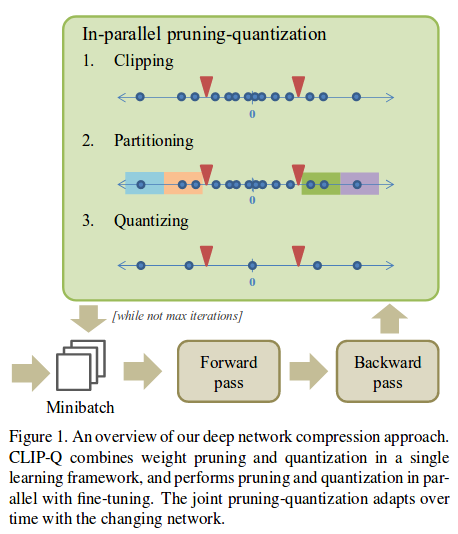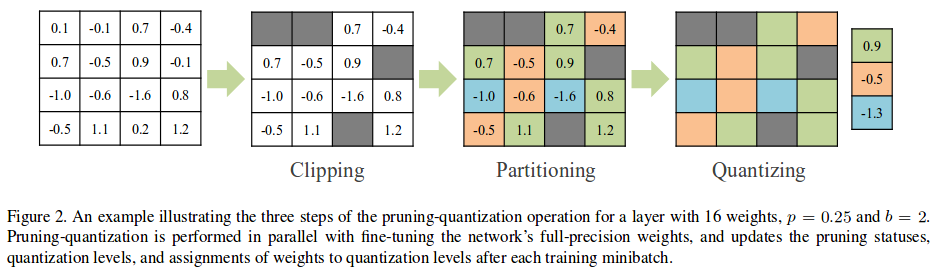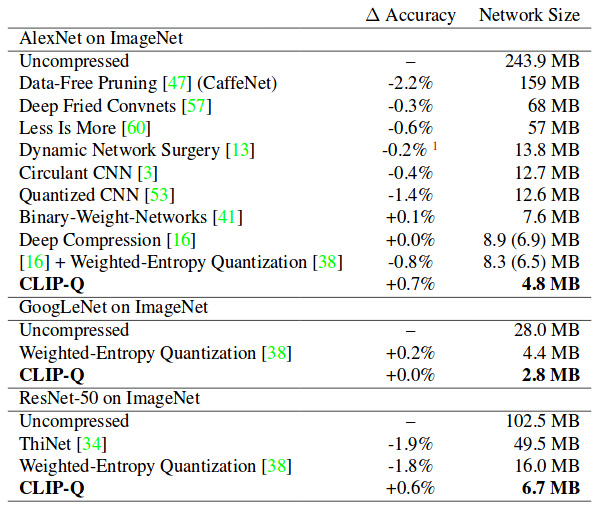The authors propose a network compression method that combines pruning and quantization.

• They use unstructured pruning, and do not care about acceleration; they only measure the storage requirements of the weight tensors.
• They use a standard sparse coding scheme to acheive practical space savings after quantizing.
• They show strong results.# Method1. Clipping: Set two thresholds $$c^-$$ and $$c^+$$ so that a fraction $$p$$ of all weights are between these thresholds. For the next forward pass, these weights will be set to zero. In the next forward pass, they may be non-zero. $$p$$ is constant, but $$c^-$$ and $$c^+$$ change.

2. Partitionning: Divide the non-clipped portion of the axis of weight values into $$2^b-1$$ intervals, where $$b$$ is the bits budget.

3. Quantizing: Quantize the weights to the average of their interval (for the next forward pass only).

# Setting hyperparameters

Prior to running Clip-Q, we need to find $$p$$ and $$b$$. The authors use Bayesian optimization; more specifically, they use a Gaussian Process. They use this method for each layer, and find $$\theta_i = (p_i, b_i)$$ for each layer $$i$$. The optimze the following objective:

$\mathrm{min}_{\theta} \quad \epsilon(\theta) - \lambda * c_i(\theta)$

where $$\epsilon(\theta)$$ is the error on a subset of the training set and $$c_i(\theta)$$ is a measure of compression benefit (economy of bits). See section 3.2 of the paper for more details.

# Results